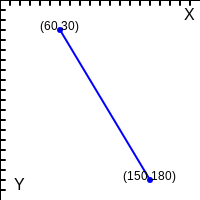# OE2DPoint¶

class OE2DPoint


This class represents OE2DPoint that stores an x (horizontal), y (vertical) coordinate pair.

The coordinate system used in OEDepict TK has the origin (x=0.0, y=0.0) at the top/left with the x-axis pointing to the right and the y-axis pointing down. See Figure: 2D coordinate system.2D coordinate system (X = horizontal, Y = vertical)

## Constructors¶

OE2DPoint()


Default constructor that initializes an OE2DPoint object with x = 0.0, y = 0.0 coordinates.

OE2DPoint(double x, double y)


Constructor that initializes an OE2DPoint object with the give x, y coordinates.

OE2DPoint(const OE2DPoint &rhs)


Copy constructor.

## operator=¶

OE2DPoint &operator=(const OE2DPoint &rhs)


Assignment operator.

## operator+¶

OE2DPoint operator+(const OE2DPoint &rhs) const


Addition operator: $$(a_x, a_y) + (b_x, b_y) = (a_x + b_x, a_y + b_y)$$

## operator-¶

OE2DPoint operator-(const OE2DPoint &rhs) const


Subtraction operator: $$(a_x, a_y) - (b_x, b_y) = (a_x - b_x, a_y - b_y)$$

## operator/¶

OE2DPoint operator/(double) const


Division operator: $$(a_x, a_y) / D = (a_x / D, a_y / D)$$

## operator *¶

OE2DPoint operator *(double) const


Multiplication operator: $$(a_x, a_y) * D = (a_x * D, a_y * D)$$

## operator+=¶

OE2DPoint &operator+=(const OE2DPoint &p)


Addition assignment operator.

## operator-=¶

OE2DPoint &operator-=(const OE2DPoint &p)


Subtraction assignment operator.

## operator/=¶

OE2DPoint &operator/=(double)


Division assignment operator.

## operator*=¶

OE2DPoint &operator *=(double)


Multiplication assignment operator.

## GetX¶

double GetX() const


Returns the x (horizontal) coordinate of the OE2DPoint object.

See also

## GetY¶

double GetY() const


Returns the y (vertical) coordinate of the OE2DPoint object.

See also

## SetX¶

void SetX(double x)


Sets the x (horizontal) coordinate of the OE2DPoint object.

See also

## SetY¶

void SetY(double y)


Sets the y (vertical) coordinate of the OE2DPoint object.

See also